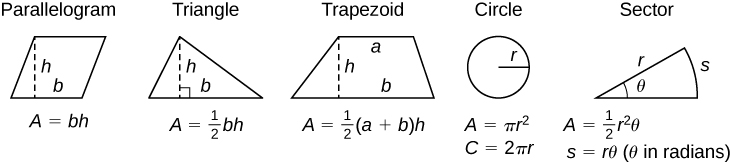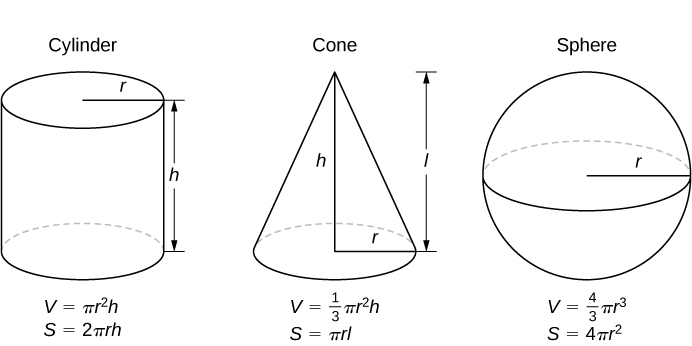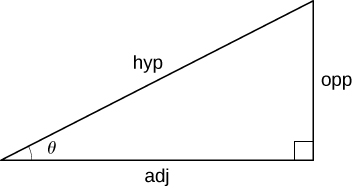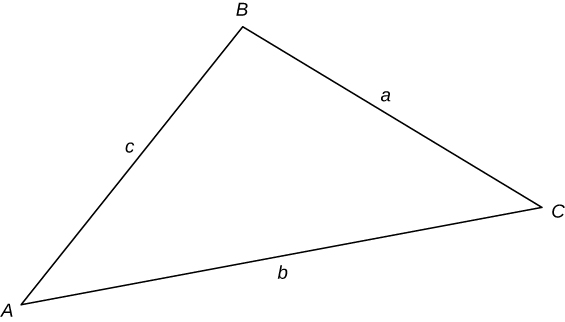# 0.3 Review of pre-calculus

 Page 1 / 1

## Formulas from geometry

$A=\text{area},$ $V=\text{Volume},\phantom{\rule{0.2em}{0ex}}\text{and}$ $S=\text{lateral surface area}$## Laws of exponents

$\begin{array}{ccccccccccccc}\hfill {x}^{m}{x}^{n}& =\hfill & {x}^{m+n}\hfill & & & \hfill \frac{{x}^{m}}{{x}^{n}}& =\hfill & {x}^{m-n}\hfill & & & \hfill {\left({x}^{m}\right)}^{n}& =\hfill & {x}^{mn}\hfill \\ \hfill {x}^{\text{−}n}& =\hfill & \frac{1}{{x}^{n}}\hfill & & & \hfill {\left(xy\right)}^{n}& =\hfill & {x}^{n}{y}^{n}\hfill & & & \hfill {\left(\frac{x}{y}\right)}^{n}& =\hfill & \frac{{x}^{n}}{{y}^{n}}\hfill \\ \hfill {x}^{1\text{/}n}& =\hfill & \sqrt[n]{x}\hfill & & & \hfill \sqrt[n]{xy}& =\hfill & \sqrt[n]{x}\sqrt[n]{y}\hfill & & & \hfill \sqrt[n]{\frac{x}{y}}& =\hfill & \frac{\sqrt[n]{x}}{\sqrt[n]{y}}\hfill \\ \hfill {x}^{m\text{/}n}& =\hfill & \sqrt[n]{{x}^{m}}={\left(\sqrt[n]{x}\right)}^{m}\hfill & & & & & & & & & & \end{array}$

## Special factorizations

$\begin{array}{ccc}\hfill {x}^{2}-{y}^{2}& =\hfill & \left(x+y\right)\left(x-y\right)\hfill \\ \hfill {x}^{3}+{y}^{3}& =\hfill & \left(x+y\right)\left({x}^{2}-xy+{y}^{2}\right)\hfill \\ \hfill {x}^{3}-{y}^{3}& =\hfill & \left(x-y\right)\left({x}^{2}+xy+{y}^{2}\right)\hfill \end{array}$

If $a{x}^{2}+bx+c=0,$ then $x=\frac{\text{−}b±\sqrt{{b}^{2}-4ca}}{2a}.$

## Binomial theorem

${\left(a+b\right)}^{n}={a}^{n}+\left(\begin{array}{l}n\\ 1\end{array}\right){a}^{n-1}b+\left(\begin{array}{l}n\\ 2\end{array}\right){a}^{n-2}{b}^{2}+\cdots +\left(\begin{array}{c}n\\ n-1\end{array}\right)a{b}^{n-1}+{b}^{n},$

where $\left(\begin{array}{l}n\\ k\end{array}\right)=\frac{n\left(n-1\right)\left(n-2\right)\cdots \left(n-k+1\right)}{k\left(k-1\right)\left(k-2\right)\cdots 3\cdot 2\cdot 1}=\frac{n!}{k!\left(n-k\right)!}$

## Right-angle trigonometry

$\begin{array}{cccc}\text{sin}\phantom{\rule{0.1em}{0ex}}\theta =\frac{\text{opp}}{\text{hyp}}\hfill & & & \text{csc}\phantom{\rule{0.1em}{0ex}}\theta =\frac{\text{hyp}}{\text{opp}}\hfill \\ \text{cos}\phantom{\rule{0.1em}{0ex}}\theta =\frac{\text{adj}}{\text{hyp}}\hfill & & & \text{sec}\phantom{\rule{0.1em}{0ex}}\theta =\frac{\text{hyp}}{\text{adj}}\hfill \\ \text{tan}\phantom{\rule{0.1em}{0ex}}\theta =\frac{\text{opp}}{\text{adj}}\hfill & & & \text{cot}\phantom{\rule{0.1em}{0ex}}\theta =\frac{\text{adj}}{\text{opp}}\hfill \end{array}$## Trigonometric functions of important angles

 $\theta$ $\text{Radians}$ $\text{sin}\phantom{\rule{0.1em}{0ex}}\theta$ $\text{cos}\phantom{\rule{0.1em}{0ex}}\theta$ $\text{tan}\phantom{\rule{0.1em}{0ex}}\theta$ $0\text{°}$ $0$ $0$ $1$ $0$ $30\text{°}$ $\text{π}\text{/}\text{6}$ $1\text{/}2$ $\sqrt{3}\text{/}2$ $\sqrt{3}\text{/}3$ $45\text{°}$ $\text{π}\text{/}\text{4}$ $\sqrt{2}\text{/}2$ $\sqrt{2}\text{/}2$ $1$ $60\text{°}$ $\text{π}\text{/}\text{3}$ $\sqrt{3}\text{/}2$ $1\text{/}2$ $\sqrt{3}$ $90\text{°}$ $\text{π}\text{/}2$ $1$ $0$ —

## Fundamental identities

$\begin{array}{cccccccc}\hfill {\text{sin}}^{2}\theta +{\text{cos}}^{2}\theta & =\hfill & 1\hfill & & & \hfill \text{sin}\left(\text{−}\phantom{\rule{0.1em}{0ex}}\theta \right)& =\hfill & \text{−}\text{sin}\phantom{\rule{0.1em}{0ex}}\theta \hfill \\ \hfill 1+{\text{tan}}^{2}\theta & =\hfill & {\text{sec}}^{2}\theta \hfill & & & \hfill \text{cos}\left(\text{−}\phantom{\rule{0.1em}{0ex}}\theta \right)& =\hfill & \text{cos}\phantom{\rule{0.1em}{0ex}}\theta \hfill \\ \hfill 1+{\text{cot}}^{2}\theta & =\hfill & {\text{csc}}^{2}\theta \hfill & & & \hfill \text{tan}\left(\text{−}\phantom{\rule{0.1em}{0ex}}\theta \right)& =\hfill & \text{−}\text{tan}\phantom{\rule{0.1em}{0ex}}\theta \hfill \\ \hfill \text{sin}\left(\frac{\pi }{2}-\theta \right)& =\hfill & \text{cos}\phantom{\rule{0.1em}{0ex}}\theta \hfill & & & \hfill \text{sin}\left(\theta +2\pi \right)& =\hfill & \text{sin}\phantom{\rule{0.1em}{0ex}}\theta \hfill \\ \hfill \text{cos}\left(\frac{\pi }{2}-\theta \right)& =\hfill & \text{sin}\phantom{\rule{0.1em}{0ex}}\theta \hfill & & & \hfill \text{cos}\left(\theta +2\pi \right)& =\hfill & \text{cos}\phantom{\rule{0.1em}{0ex}}\theta \hfill \\ \hfill \text{tan}\left(\frac{\pi }{2}-\theta \right)& =\hfill & \text{cot}\phantom{\rule{0.1em}{0ex}}\theta \hfill & & & \hfill \text{tan}\left(\theta +\pi \right)& =\hfill & \text{tan}\phantom{\rule{0.1em}{0ex}}\theta \hfill \end{array}$

## Law of sines

$\frac{\text{sin}\phantom{\rule{0.1em}{0ex}}A}{a}=\frac{\text{sin}\phantom{\rule{0.1em}{0ex}}B}{b}=\frac{\text{sin}\phantom{\rule{0.1em}{0ex}}C}{c}$## Law of cosines

$\begin{array}{ccc}\hfill {a}^{2}& =\hfill & {b}^{2}+{c}^{2}-2bc\phantom{\rule{0.2em}{0ex}}\text{cos}\phantom{\rule{0.2em}{0ex}}A\hfill \\ \hfill {b}^{2}& =\hfill & {a}^{2}+{c}^{2}-2ac\phantom{\rule{0.2em}{0ex}}\text{cos}\phantom{\rule{0.2em}{0ex}}B\hfill \\ \hfill {c}^{2}& =\hfill & {a}^{2}+{b}^{2}-2ab\phantom{\rule{0.2em}{0ex}}\text{cos}\phantom{\rule{0.2em}{0ex}}C\hfill \end{array}$

$\begin{array}{ccc}\hfill \text{sin}\phantom{\rule{0.2em}{0ex}}\left(x+y\right)& =\hfill & \text{sin}\phantom{\rule{0.2em}{0ex}}x\phantom{\rule{0.2em}{0ex}}\text{cos}\phantom{\rule{0.2em}{0ex}}y+\text{cos}\phantom{\rule{0.2em}{0ex}}x\phantom{\rule{0.2em}{0ex}}\text{sin}\phantom{\rule{0.2em}{0ex}}y\hfill \\ \hfill \text{sin}\phantom{\rule{0.1em}{0ex}}\left(x-y\right)& =\hfill & \text{sin}\phantom{\rule{0.2em}{0ex}}x\phantom{\rule{0.2em}{0ex}}\text{cos}\phantom{\rule{0.2em}{0ex}}y-\text{cos}\phantom{\rule{0.2em}{0ex}}x\phantom{\rule{0.2em}{0ex}}\text{sin}\phantom{\rule{0.2em}{0ex}}y\hfill \\ \hfill \text{cos}\phantom{\rule{0.1em}{0ex}}\left(x+y\right)& =\hfill & \text{cos}\phantom{\rule{0.2em}{0ex}}x\phantom{\rule{0.2em}{0ex}}\text{cos}\phantom{\rule{0.2em}{0ex}}y-\text{sin}\phantom{\rule{0.2em}{0ex}}x\phantom{\rule{0.2em}{0ex}}\text{sin}\phantom{\rule{0.2em}{0ex}}y\hfill \\ \hfill \text{cos}\phantom{\rule{0.1em}{0ex}}\left(x-y\right)& =\hfill & \text{cos}\phantom{\rule{0.2em}{0ex}}x\phantom{\rule{0.2em}{0ex}}\text{cos}\phantom{\rule{0.2em}{0ex}}y+\text{sin}\phantom{\rule{0.2em}{0ex}}x\phantom{\rule{0.2em}{0ex}}\text{sin}\phantom{\rule{0.2em}{0ex}}y\hfill \\ \hfill \text{tan}\phantom{\rule{0.1em}{0ex}}\left(x+y\right)& =\hfill & \frac{\text{tan}\phantom{\rule{0.2em}{0ex}}x+\text{tan}y}{1-\text{tan}\phantom{\rule{0.2em}{0ex}}x\phantom{\rule{0.2em}{0ex}}\text{tan}y}\hfill \\ \hfill \text{tan}\left(x-y\right)& =\hfill & \frac{\text{tan}\phantom{\rule{0.2em}{0ex}}x-\text{tan}y}{1+\text{tan}\phantom{\rule{0.2em}{0ex}}x\phantom{\rule{0.2em}{0ex}}\text{tan}y}\hfill \end{array}$

## Double-angle formulas

$\begin{array}{ccc}\hfill \text{sin}\phantom{\rule{0.2em}{0ex}}2x& =\hfill & 2\phantom{\rule{0.1em}{0ex}}\text{sin}\phantom{\rule{0.2em}{0ex}}x\phantom{\rule{0.2em}{0ex}}\text{cos}\phantom{\rule{0.2em}{0ex}}x\hfill \\ \hfill \text{cos}\phantom{\rule{0.2em}{0ex}}2x& =\hfill & {\text{cos}}^{2}x-{\text{sin}}^{2}x=2\phantom{\rule{0.1em}{0ex}}{\text{cos}}^{2}x-1=1-2\phantom{\rule{0.1em}{0ex}}{\text{sin}}^{2}x\hfill \\ \hfill \text{tan}\phantom{\rule{0.2em}{0ex}}2x& =\hfill & \frac{2\phantom{\rule{0.1em}{0ex}}\text{tan}\phantom{\rule{0.2em}{0ex}}x}{1-{\text{tan}}^{2}x}\hfill \end{array}$

## Half-angle formulas

$\begin{array}{ccc}\hfill {\text{sin}}^{2}x& =\hfill & \frac{1-\text{cos}\phantom{\rule{0.2em}{0ex}}2x}{2}\hfill \\ \hfill {\text{cos}}^{2}x& =\hfill & \frac{1+\text{cos}\phantom{\rule{0.2em}{0ex}}2x}{2}\hfill \end{array}$

Approximate root 4 without a calculator
Approximate root 4.02 without using a calculator
Tinkeu
2.03
temigbe
find the integral of tan
Differentiate each from the first principle. y=x,y=1/x
I need help with calculus. Anyone help me.
yes
Hi
Usman
beautiful name usman
Fund
really
Usman
Hi guys
Macquitasha
Hello everyone here
abdulazeez
good day!
joel
hii
Shreya
You are welcome
abdulazeez
shreya
ashif
thanks
joel
hello Sar aapse Kuchh calculate ke sawal poochhne Hain
Sumit
integration seems interesting
it's like a multiple oparation in just one.
Efrain
Definitely integration
tangent line at a point/range on a function f(x) making f'(x)
Luis
Principles of definite integration?
ROHIT
For tangent they'll usually give an x='s value. In that case, solve for y, keep the ordered pair. then find f(x) prime. plug the given x value into the prime and the solution is the slope of the tangent line. Plug the ordered pair into the derived function in y=mx+b format as x and y to solve for B
Anastasia
parcing an area trough a function f(x)
Efrain
Find the length of the arc y = x^2 over 3 when x = 0 and x = 2.
integrate x ln dx from 1 to e
application of function
how i can need help
what ?
Bunyim
defination of math
azam
application of function
azam
azam
what is a circle
Ronnie
math is the science, logic, shape and arrangement
a circle is a hole shape
Jianna
a whole circumference have equal distance from one point
azam
please tell me books which write on function
azam
HE is a Nigerian, wrote the book INTEGRATED MATHEMATICS...CHECK IT OUT!!
Agboke
Woah this is working again
Bruce
A circle is any geometric shape which has infinite lines of symmetry
Tinkeu
show that the f^n f(x)=|x-1| is not differentiable at x=1.
is there any solution manual to calculuse 1 for Gilbert Strang ?
I am beginner
Abdul
I am a beginner
ephraim
l am also beginner
just began, bois!!
Luis
Hello
abdulazeez
abdulazeez
Hey
Bonface
Hi
Jianna
what is mathematics
logical usage of numbers
Leo
thanks
Henry
you welcome
Leo
what's career can one specialize in by doing pure maths
Lucy
Lucy Omollo...... The World is Yours by specializing in pure math. Analytics, Financial engineering ,programming, education, combinatorial mathematics, Game Theory. your skill-set will be like water a necessary element of survival.
David
***onetonline.org/find/descriptor/result/1.A.1.c.1
Bruce
mathematics seems to be anthropocentric deductive reasoning and a little high order logic. I only say this because I can only find two things going on which is infinitely smaller than 0 and anything over 1
David
More comprehensive list here: ***onetonline.org/find/descriptor/result/1.A.1.c.1?a=1
Bruce
so how can we differentiate inductive reasoning and deductive reasoning
Henry
thanks very much Mr David
Henry
hi everyone
Sabir
is there anyone who can guide me in learning the mathematics easily
Sabir
Hi Sabir first step of learning mathematics is by falling in love with it and secondly, watch videos on simple algebra then read and solved problems on it
Leo
yes sabir just do every time practice that is the solution
Henry
it will be work over to you ,u know how mind work ,it prossed the information easily when u are practising regularly
Henry
in calculas,does a self inverse function exist
Lucy
I'm lost in all functions need help
Jonathan
hello i need help in rate of change
Moises
***questioncove.com/invite/QzOQGp
Bruce
Hello
hassan
hi
MJ
hi
Masaniel
so difficult
Masaniel
hello my name is Charles Christian
Hello Charles
Jianna
Hi! I am Dante
Dante
Hi! I'm ashwini
ashwini
halĺo
Roben
Hi
Leo
hello leo
Agboke
can anyone prove why AU(BnC)=(AUB)n(AUC)
Agboke
this one it can't be proven these are assumption
Henry
hello agboke there is no proof for such
Leo
Hi
hi this is wasim
wasim
can anybody put me through flowchart and algorithm here
Agboke
Leo
Luis
music while you math
LuisByByByBy Abishek DevarajBy